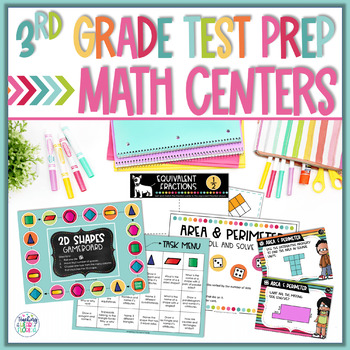# Math Centers | Math Test Prep | 3rd Grade Math Spiral Review

Rated 5 out of 5, based on 1 reviews
1 Rating;
3rd
Subjects
Resource Type
Standards
Formats Included
• Zip
Pages
147 pages
\$13.00
List Price:
\$20.00
You Save:
\$7.00
\$13.00
List Price:
\$20.00
You Save:
\$7.00
Report this resource to TPT

### Description

With 3rd-grade math test prep season around the corner, it can be challenging to help your students gain that extra edge to feel confident and ready for the big day. Luckily, you don't need to plan everything out yourself - these PRINT & GO math centers have fresh content and activities specifically tailored to 3rd-grade topics like 2D Shapes, Area & Perimeter, Data Analysis, Division Fact Fluency, Elapsed Time, Equivalent Fractions, Multiplication Fact Fluency, Place Value, Rounding, and Word Problems.

Reviewing essential 3rd-grade math skills before the BIG Test each year is time well spent! With these math centers at your side, you can easily engage your students in targeted practice so they can confidently tackle the test.

───────────────────────────────

⭐ Click on the PREVIEW to take a closer look!

───────────────────────────────

Included in this 3rd Grade Math Test Prep Math Centers Resource:

◻️ 2D Shapes: Naming and categorizing quadrilaterals

◻️ Area & Perimeter: measuring area and perimeter, finding unknown sides

◻️ Data Analysis: reading and analyzing bar graphs, pictographs, data charts, and plot maps

◻️ Division Fact Fluency: Mastering division facts with timed practice

◻️ Elapsed Time: Telling time, finding elapsed time, using number lines

◻️ Equivalent Fractions: Sorting and matching equivalent fractions

◻️ Multiplication Fact Fluency: Mastering multiplication facts with timed practice

◻️ Place Value: Valuable review of place value concepts for 2, 3, and 4 digit numbers

◻️ Rounding to the Nearest 10 and 100: Using place value understanding to round 2, 3, and 4 digit numbers

◻️ Word Problems: Solving one and two-step word problems for 2D Shapes, Area & Perimeter, Addition, Data Analysis, Division, Elaped Time, Equivalent Fractions, Comparing Fractions, Measurement, Multiplication, Place Value, & Rounding.

◻️ Student Recording Sheets

───────────────────────────────

Need more test prep resources?

───────────────────────────────

"Don't forget to leave feedback -you will receive TPT credits that you can use toward future purchases!

Thank you for visiting Teaching in the Heart of Florida!

Total Pages
147 pages
Included
Teaching Duration
2 Weeks
Report this resource to TPT
Reported resources will be reviewed by our team. Report this resource to let us know if this resource violates TPT’s content guidelines.

### Standards

to see state-specific standards (only available in the US).
Tell and write time to the nearest minute and measure time intervals in minutes. Solve word problems involving addition and subtraction of time intervals in minutes, e.g., by representing the problem on a number line diagram.
Measure and estimate liquid volumes and masses of objects using standard units of grams (g), kilograms (kg), and liters (l). Add, subtract, multiply, or divide to solve one-step word problems involving masses or volumes that are given in the same units, e.g., by using drawings (such as a beaker with a measurement scale) to represent the problem.
Draw a scaled picture graph and a scaled bar graph to represent a data set with several categories. Solve one- and two-step “how many more” and “how many less” problems using information presented in scaled bar graphs. For example, draw a bar graph in which each square in the bar graph might represent 5 pets.
Recognize area as an attribute of plane figures and understand concepts of area measurement.
A square with side length 1 unit, called “a unit square,” is said to have “one square unit” of area, and can be used to measure area.